#### Example Programs

Program 

Write a program to input 10 integers and find the sum of the prime numbers only.

Program 

Write a program to input 10 integers and find the average of the prime numbers only.

Program 

Write a program to input 10 integers and find the sum of the perfect numbers only.

Program 

Write a program to input 10 integers and find the average of the perfect numbers only.

Program 

Write a program to input 10 integers and find the largest prime number if any.

Program 

Write a program to input 10 integers and find the smallest perfect number if any.

Program 

Write a program to generate all 2 digit prime numbers.

Program 

Write a program to generate all perfect numbers between 1 to 100.

Program 

Write a program to generate all 3 digit palindrome numbers.

Program 

WAP to Input an integer and check whether it is a special number or not.

A special number is such a number whose sum of the factorials of each digit is the number itself. For example, 145 is a special number because 1! + 4! + 5! = 1 + 24 +120 = 145. Special numbers are also called factorion.

Program 

Write a program to input a number and check whether it is a magic number or not. If you iterate the process of summing the squares of the decimal digits of the number, and if this process terminates in 1, then the original number is called a magic number. For example 55=> (5+5)=10=>(1 +0)=1.

Program 

Write a program to input an integer as argument, and print the number by having the digits arranged in ascending order.

Program 

Write a program to pass an integer as argument, and check whether all digits in it are unique or not.

Program 

Write a program to pass an integer as argument, and print the frequency of each digit in it.

Program 

Write a program to input 10 numbers and print the largest prime number if any.

Program 

Write a program to generate all 2 digit twisted prime number. Twisted prime number is a number which is prime and its reverse is also prime. Example 13 and 31.

Program 

WAP to Input an integer and form a new number where the digits are in ascending order.

Program 

Write a program to pass an integer as argument and print the second largest digit

Program 

Write a program to print Floyd's triangle as shown below:

1

2  3

4  5  6

7  8  9  10

11 12 13 14 15

Program 

Write a Java program to print Following Pattern: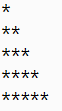Program 

Write a Java program to print Following Pattern: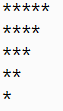Program 

Write a Java program to print Following Pattern: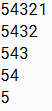Program 

Write a Java program to print Following Pattern: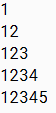Program 

Write a Java program to print Following Pattern: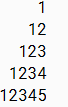Program 

Write a Java program to print Following Pattern: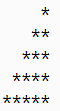Program 

Write a Java program to print Following Pattern: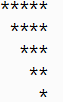Program 

Write a program in java to print all prime Palindrome numbers between 'm' and 'n'

Sample Example:

Enter the value of m :

1

Enter the value of n :

1000

Prime Palindrome number between 1 and 1000 are:

2 3 5 7 11 101 131 151 181 191 313 353 373 383 727 757 787 797 919 929

Solution 1:

`import java.util.*;class PrimePalindrome{  public static void main(String arr[])  {      Scanner sc=new Scanner(System.in);      int m,n,i,a,digit,rev;      boolean flag;      System.out.println("Enter the value of m :");      m=sc.nextInt();      System.out.println("Enter the value of n :");      n=sc.nextInt();      System.out.println("Prime Palindrome number between "+m+" and "+n+" are: ");      for(i=m;i<=n;i++)      {          a=i;          rev=0;          while(a>0)          {              digit=a%10;              rev=rev*10+digit;              a=a/10;          }          if(i==rev)          {              flag=true;              for(a=2;a<i;a++)              {                  if(i%a==0)                  {                      flag=false;                      break;                  }              }              if(flag==true&&i>1)              {                  System.out.print(i+" ");               }          }      }  }}`

Solution 2: Using Function

`import java.util.*;class PrimePalindrome{  boolean isPrime(int n)  {      int a;      for(a=2;a<=n/2;a++)      {          if(n%a==0)            return false;      }      if(n==1)        return false;      else        return true;  }  boolean isPalindrome(int n)  {      int rev=0,t;      t=n;      while(t>0)      {          rev=rev*10+(t%10);          t=t/10;      }      if(rev==n)                return true;      else        return false;  }  public static void main(String arr[])  {      Scanner sc=new Scanner(System.in);      int m,n,i,a,digit,rev;      PrimePalindrome obj=new PrimePalindrome();      boolean flag;      System.out.println("Enter the value of m :");      m=sc.nextInt();      System.out.println("Enter the value of n :");      n=sc.nextInt();      System.out.println("Prime Palindrome number between "+m+" and "+n+" are: ");      for(i=m;i<=n;i++)      {          if(obj.isPalindrome(i)&&obj.isPrime(i))          {                System.out.print(i+" ");          }                }  }}`